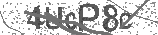﻿ Adding & Subtracting Fractions Song: LIKE and UNLIKE Denominators | Safe Videos for Kids
Welcome

# Adding & Subtracting Fractions Song: LIKE and UNLIKE Denominators

Thanks! Share it with your friends!

URL

You disliked this video. Thanks for the feedback!

Sorry, only registred users can create playlists.URLFind Related Videos  added
562 Views## Description

Enjoy our growing library of math videos at https://www.numberock.com ◀ SAFE LINK

NUMBEROCK DIGITAL LIBRARY: https://www.teacherspayteachers.com/Product/Math-Worksheets-More-NUMBEROCK-Musical-Math-Workbook-and-Activities-2200780 ◀ Safe Link

In a future time, when AI robots have taken over running pizzerias on a planet orbiting Alpha Centauri, the robot Zigon takes orders and then adds and subtracts fractions before the Pizzamatic-5000 serves up large pepperoni and cheese pizzas for its alien visitors!

UK Equivalent
Year 5 - Year 6 - Year 7 - Maths

Lyrics:
This is how the least common multiple’s found:
write a list of each denominator’s multiples down;
the smallest number you can find in both of them
is the least common multiple (a.k.a. the LCM.)

The least common multiple can also be
called the least common denominator - or LCD.
It stays the same in the difference or the sum
when you subtract or add two fractions.

Three-sixths of a pizza minus one-fourth:
find the LCM, which is twelve, of course.
(Twelve is the least common denominator.)
Make equivalent fractions (six-twelfths and three-twelfths).
Then, do the subtraction: six minus three is three...
three-twelfths of a pizza at the pizzeria.

Chorus:
Find the least common multiple; that’s the first action.
Next, is finding equivalent fractions.
Then, do the addition or subtraction.
Simplify like an automatic reaction.

Two-fourths of a pizza plus one-fifth:
it smelled so good we had to give it a sniff.
The LCM was twenty (the new denominator).
We found equivalents quickly: ten-twentieths plus four-twentieths... fourteen-twentieths of a pizza at the pizzeria.
(Chorus)
Five-sixths of a pizza minus one-third:
I knew the LCM was six, and have you heard?
It’s also called the least common denominator.
I found equivalent fractions (five-sixths and two-sixths).
Then, I did the subtraction: five minus two is three...
three-sixths of a pizza at the pizzeria.
(Chorus)

Common Core Standards Covered in This Song:

5.NF.A

5.NF.A.1 - Add and subtract fractions with unlike denominators (including mixed numbers) by replacing given fractions with equivalent fractions in such a way as to produce an equivalent sum or difference of fractions with like denominators.

5.NF.A.2 - Solve word problems involving addition and subtraction of fractions referring to the same whole, including cases of unlike denominators, e.g., by using visual fraction models or equations to represent the problem. Use benchmark fractions and number sense of fractions to estimate mentally and assess the reasonableness of answers.

4.NF.B

4.NF.B.3 D - Solve word problems involving addition and subtraction of fractions referring to the same whole and having like denominators, e.g., by using visual fraction models and equations to represent the problem.

4.NF.B.3 A - Understand addition and subtraction of fractions as joining and separating parts referring to the same whole.

3.NF.A

3.NF.A.3 - Understand two fractions as equivalent (equal) if they are the same size, or the same point on a number line.

4.NF.A

4.NF.A.1 - Explain why a fraction a/b is equivalent to a fraction (n × a)/(n × b) by using visual fraction models, with attention to how the number and size of the parts differ even though the two fractions themselves are the same size. Use this principle to recognize and generate equivalent fractions.

http://www.NUMBEROCK.com is a provider of educational videos for kids which was founded by an innovative 5th Grade teacher who envisioned a new kind of classroom where students got energized for math class. Parents of his students even noticed their children singing mathematics songs around the dinner table well beyond school hours.

Seeing his students so enthusiastic when it was time for math was welcome, to say the least! But the epiphanous moment was when students were singing math songs all-day long like they were in the American Top 40!

We'll be releasing new songs and videos regularly until eventually addressing all the math skills and concepts that a Common Core Curriculum demands from 1st Grade to 2nd Grade, and even 5th Grade to 6th Grade.

Our main goal is to make teaching and learning math more fun and more interactive. SchoolHouse Rock! has done this in other subjects for us in the previous generations.Be the first to comment